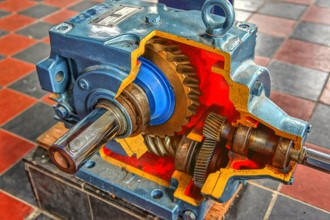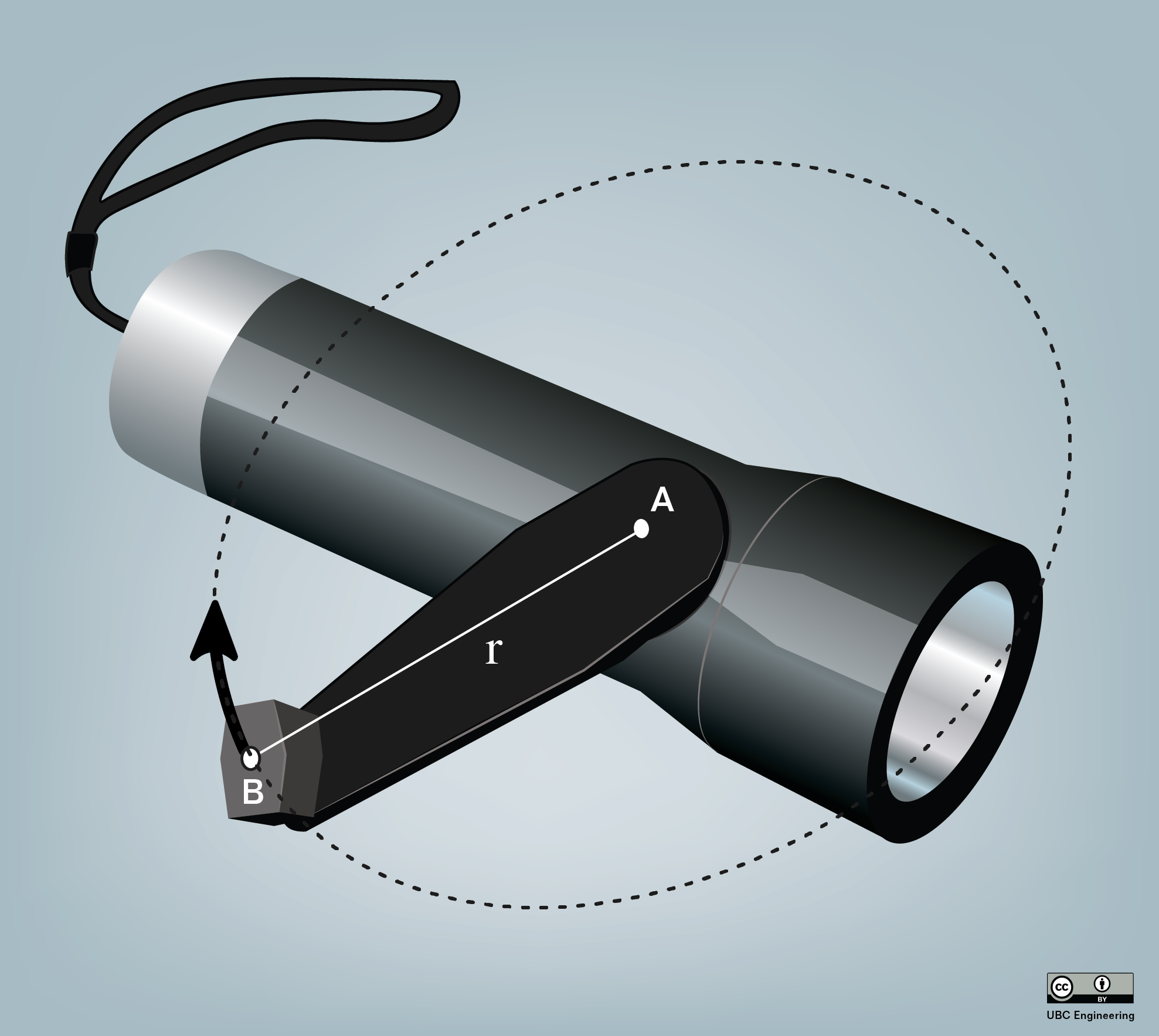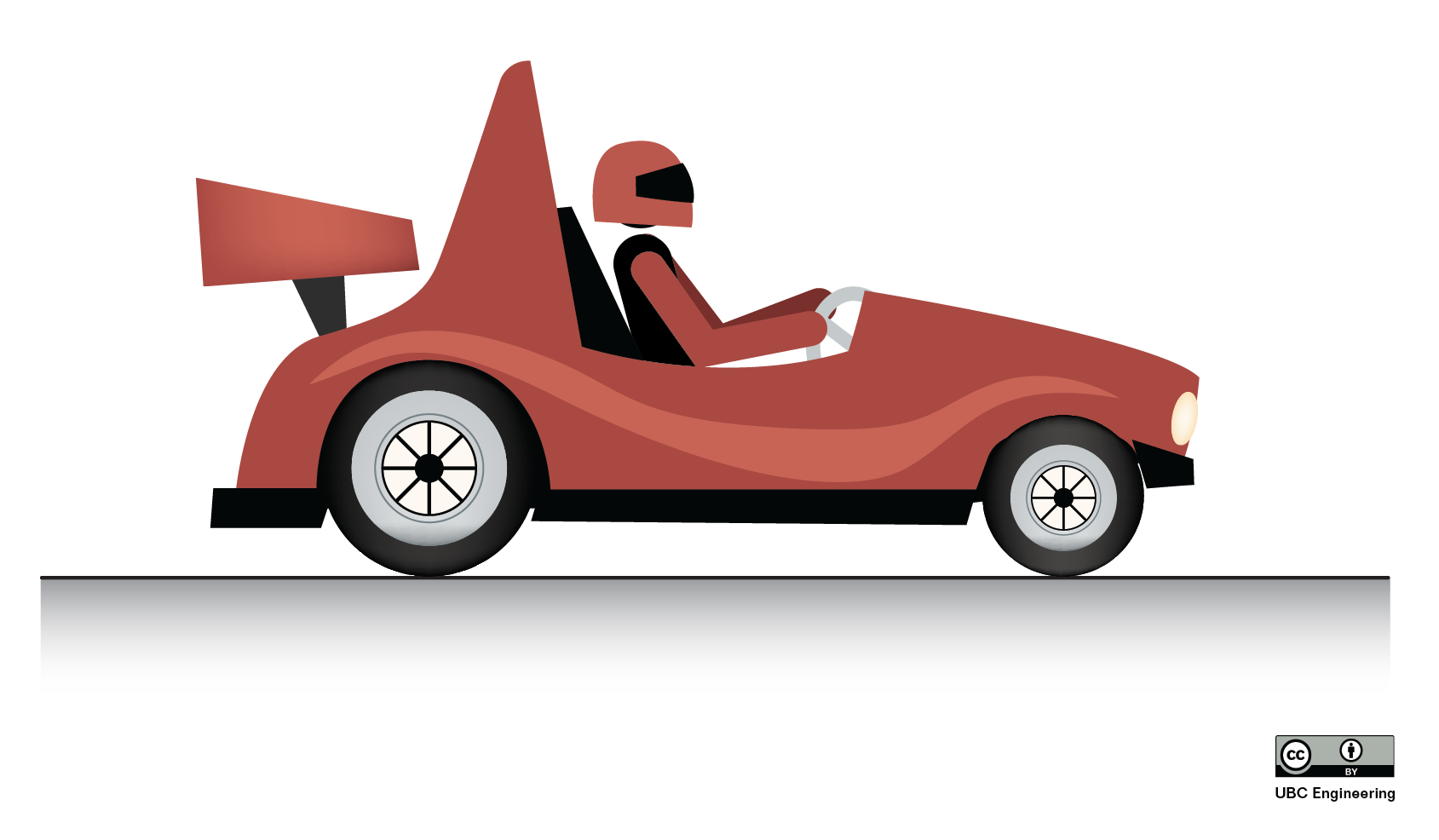﻿ Mechanics Map - Efficiency in Rigid Body Systems

# Efficiency in Rigid Body Systems

Any devices with work/power inputs and outputs will have some loss of work or power between that input and output due to inefficiencies such as friction. While energy is always conserved according to the principle of work and energy, some energies such as the heat generated via friction may not be considered useful. A measure of the useful work or power that makes it from the input of a device to the output is the efficiency. Specifically efficiency (denoted by the lowercase Greek letter eta) is defined as the useful work out of a device divided by the work into the device. With power being the work over time, efficiency can also be described as useful power out divided by the power in to a device (the time term would cancel out leaving us with our original definition).

 $\eta=\frac{W_{out}}{W_{in}}=\frac{P_{out}}{P_{in}}$

Efficiency is a unitless measure that is always between zero and one, though it may also be denoted as a percentage. The higher the efficiency, the greater the percentage of work or power that makes it from the input to the output.

Because kinematics will usually not allow for a drop in velocities or angular velocities in mechanical systems (for example the teeth in gear systems can't pass through one another), the efficiency will play out as a loss of force or moments rather than a loss of velocities.

 Translational: $F_{out}=\eta\frac{F_{in}\omega_{in}}{\omega_{out}}$ Rotational: $M_{out}=\eta\frac{M_{in}v_{in}}{v_{out}}$

It is impossible to have efficiencies greater than one (or 100%) because that would be a violation of the conservation of energy, however for most devices we wish to get the efficiencies as close to one as possible. This is not only because it wastes less work or power, but also because any work or power that is "lost" in the device will be turned into heat that may build up. If it is large enough, this heat build up may also require the addition of a cooling system to draw that heat away.

## Worked Problems:

### Question 1:

The input to a gearbox has a measured 32 foot pounds of torque at 700 rpm. The output has 207 foot pounds of torque at 100 rpm.

• What is the power at the input?
• What is the power at the output?
• What is the efficiency of the gearbox?### Question 2:

Lucy is playing with a rechargeable flashlight. Spinning the handle generates electrical energy which can be stored to power the three watt bulb. Lucy rotates the handle with a constant moment of 2 newton meters and an average angular velocity of 3 radians per second for 30 seconds. When she stops turning and switches the flashlight on the bulb stays lit for 1 second. Given this information, how efficient is the flashlight in converting mechanical energy to usable electrical energy?### Question 3:

A race car is traveling along a straight road at a constant speed of 80 kilometers per hour. The engine provides a 50 newton meter torque at each of the back two wheels (radius r = 0.5m). What is the power being applied at the wheels? What is the efficiency of the transmission if the input power from the motor is 17.78 kilowatts?